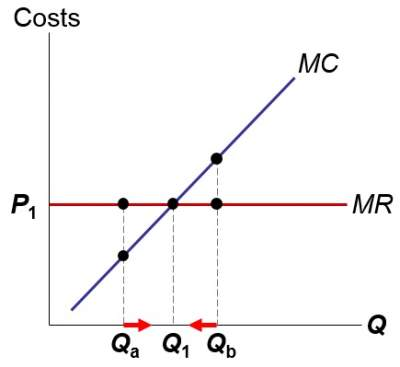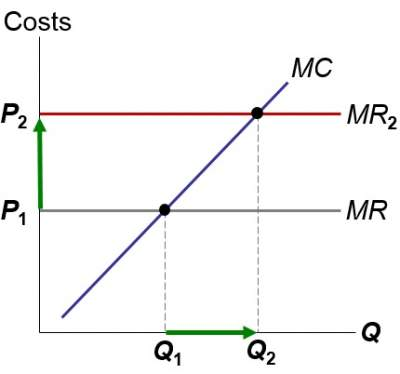# Firms in Competitive Markets

## Characteristics of Perfect Competition

The characteristics of perfect competition are as follows:
1. Many buyers and many sellers.
2. The goods offered for sale are largely the same.
3. Firms can freely enter or exit the market.
Points 1 & 2 imply that each buyer and seller is a "price taker", which means takes the price as given.

## The Revenue of a Competitive Firm

The equations for the revenue of a competitive firm are expressed as under:
• Total revenue (TR) = P × Q
• Average revenue (AR) = TR / Q = P
• Marginal revenue (MR) = ΔTR / ΔQ

### Exercise

Exercise on Revenue of a Competitive Firm

Check your answers here: Solution to the Exercise on Revenue of a Competitive Firm

Note that MR = P happens for competitive firms in competitive markets
So, a competitive firm can keep increasing its output without impacting the market price.
Hene, every unit produced (increase in Q) would in turn increase revenue by P.

## Profit Maximization

How is it possible to find Q that maximizes the firm's profit?
Here, we need to think at the margin.
Basically, if Q increases by 1 unit then revenues increases by MR and cost increases by MC.
Therefore, if MR < MC, then reduce Q to increase profit; and if MR > MC, then increase Q to increaase profit.

### Example

QTRTCProfitMRMCΔProfit = MR − MC
0 \$0 \$10 −\$10 .... .... ....
1 \$20 \$15 \$5 \$20 \$5 \$15
2 \$40 \$30 \$10 \$20 \$15 \$5
3 \$60 \$42 \$18 \$20 \$12 \$8
4 \$80 \$60 \$20 \$20 \$18 \$2
5 \$100 \$75 \$25 \$20 \$15 \$5

## MC and the Firm's Supply Decision

Rule: MR = MC at the profit-maximizing Q.
 If MC < MR at Qa. Hence increase Q to increase profit. If MC > MR at Qb. Hence increase Q to increase profit. If MC = MR at Q1. Hence changing Q would decrease profit.If price increases to P2, then the profit-maximizing quantity rises to Q2. The MC curve determines the firm's Q at any price. Hence, the MC curve is the firm's supply curve.## Exit vs Shutdown

A Firm's decision to leave (exit) the market is a long-run (LR) decision.
However, shutdown a short-run (SR) decision to stop production due to certain market conditions.
The main difference between the two is that in a shutdown situtation, the firm must pay fixed costs whereas if leaving the market then the costs are zero.
Hence, the cost of shutting down is that revenue loss = TR, and the Benefit is cost savings = VC
Using formula to express these two scenarios, decide to shutdown if TR < VC (and when dividing both side by Q), or if P < AVC

## A Firm's Decision to Exit or to Enter the Market

Exiting
• Cost of exiting the market: revenue loss = TR
• Benefit of exiting the market: cost savings = TC (zero FC in the long run)
• Hence, firm exits if TR < TC and when divide both by Q we obtain if P < ATC
Entering
• A firm will enter the market if TR > TC, similarly, if P > ATC

### Exercise

Exercise-1 on Identifying a firm's profit

Check your answers here: Solution-1 to the Exercise on Identifying a firm's profit

Exercise-2 on Identifying a firm's profit

Check your answers here: Solution-2 to the Exercise on Identifying a firm's profit

## SR Market and Entry/Exit in LR

• Due to fixed costs, the number of firms in the market is fixed in the short run.
However, this number is variable in the long run due to free entry and exit.
• During the SR market supply curve and as long as P ≥ AVC,
each firm will produce its profit-maximizing quantity, where MR = MC.
• Whenever firms keep earning and getting positive economic profits,
new firms will eventually enter the market.
• Subsequently, price and profit will decrease leading towards a slow entry.
On the other hand, if firms are experiencing losses then some of them will eventually exit the market causing price to increase and
diminish remaining firms' losses.

• ### Exercise

Exercise on Zero-Profit Condition